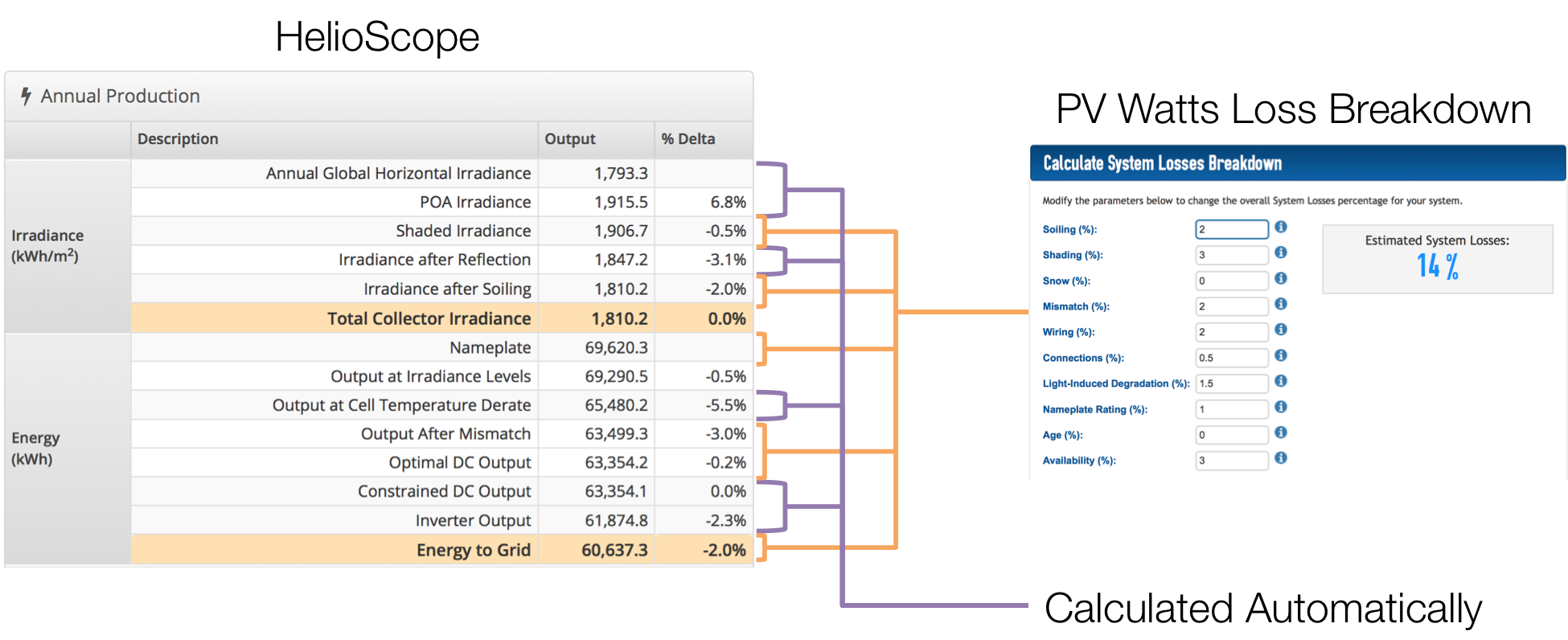# HelioScope Versus PV Watts

### Introduction

HelioScope and PVWatts are both tools used to estimate energy production of solar arrays. The two programs are similar in many ways, including most of the major elements of sun angle and system behavior calculations. Both models can be lined up to within a few percent of each other, assuming the user has selected the same weather files, system design parameters, and loss factors. However, there are some assumptions that are unique to each model, so they will likely not line up perfectly by default.

### Differences between HelioScope and PV Watts

HelioScope:

• Based on bottoms-up simulation of system performance (including module-level calculations of sunlight and module physics)
• Based on specific modules and inverters (unique efficiency and thermal behavior)
• Calculations include adjustments for low-light performance (“Output at Irradiance”), row-to-row spacing, and obstruction shading (plus any associated mismatch), which are not included in PVWatts

PV Watts:

• Top-down estimate of system production based on multiplying system nameplate
• Does not include specific module or inverter components
• Most loss factors are based on “derates” defined by the user
• Calculations include additional loss parameters for light induced degradation (default 1.5% loss) and system availability (default 3% loss)

### How to Align HelioScope & PV Watts

1. Make sure the weather files are the same. The default Condition Set in HelioScope uses the closest weather station, which is often Solar Prospector, as opposed to TMY2/TMY3 (which is what PVWatts uses). If so, create a new Condition Set with the same weather file.
2. Check components, particularly:
1. Module temperature coefficient: PV Watts assumes 0.47%/ºC losses for all standard modules, whereas HelioScope uses the specific temperature coefficients of each module
2. Inverter efficiency: PV watts assumes a standard 96% efficient inverter, HelioScope calculates inverter efficiency based on the specific efficiency of the device (typically based on specific power & voltage levels)
3. System Design: Make sure the design assumptions (including racking type, module tilt & azimuth, DC/AC ratio) are the same
4. Adjust HelioScope losses to account for additional loss parameter defaults in PV Watts: light induced degradation (1.5%) can be added to soiling losses, and availability (default 3% in PVWatts) can be added to AC losses

### Mapping the Loss Table to PV Watts

When looking at the HelioScope loss table in comparison to the PV Watts assumptions, keep the following in mind:

• Irradiance calculations, reflection losses, and temperature losses are calculated, but not shown, in PV Watts
• About half of the lines on the HelioScope loss table line are mapped to the PV Watts Loss Breakdown. See Figure 1 below for more detail.Figure 1: Mapping from HelioScope Loss Table to PV Watts Loss Breakdown